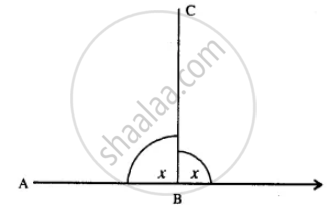# Find the Number of Sides in a Regular Polygon, If Its Interior Angle is Equal to Its Exterior Angle. - Mathematics

Sum

Find the number of sides in a regular polygon, if its interior angle is equal to its exterior angle.

#### Solution

Let each exterior angle or interior angle be = x°∴ x + x = 180°

2x = 180°

x = 90°

Now, let no. of sides = n

∵ each exterior angle = 360^circ/"n"

∴ 90° = 360^circ/"n"

n = 360^circ/90^circ

n = 4

Concept: Regular Polynomial
Is there an error in this question or solution?

#### APPEARS IN

Selina Concise Mathematics Class 8 ICSE
Chapter 16 Understanding Shapes
Exercise 16 (B) | Q 6 | Page 184